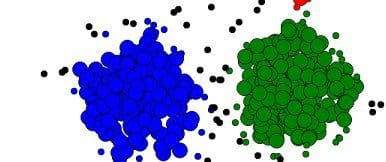# Comparing Clustering Techniques: A Concise Technical Overview

A wide array of clustering techniques are in use today. Given the widespread use of clustering in everyday data mining, this post provides a concise technical overview of 2 such exemplar techniques.

Clustering is used for analyzing data which does not include pre-labeled classes. Data instances are grouped together using the concept of maximizing intraclass similarity and minimizing the similarity between differing classes. This translates to the clustering algorithm identifying and grouping instances which are very similar, as opposed to ungrouped instances which are much less-similar to one another. As clustering does not require the pre-labeling of classes, it is a form of unsupervised learning.k-means Clustering is perhaps the most well-known example of a clustering algorithm. However, it is not the only one. Completely different schemes exist, such hierarchical clustering, fuzzy clustering, and density clustering, as do different takes on centroid-style clustering, such as using the median (as opposed to the mean), or ensuring that the centroid is a cluster member (read on for some context).

Below is a brief technical overview of the k-means clustering algorithm, as well as Expectation-Maximization (EM), a Gaussian distribution-based clustering method. Both approaches attempt to maximize intraclass similarity and minimize inter-class similarity, yet take different approaches in doing so.

k-means Clustering

k-means is a simple, yet often effective, approach to clustering. k points are randomly chosen as cluster centers, or centroids, and all training instances are plotted and added to the closest cluster. After all instances have been added to clusters, the centroids, representing the mean of the instances of each cluster are re-calculated, with these re-calculated centroids becoming the new centers of their respective clusters.

At this point, all cluster membership is reset, and all instances of the training set are re-plotted and -added to their closest, possibly re-centered, cluster. This iterative process continues until there is no change to the centroids or their membership, and the clusters are considered settled.Fig.1: k-means Clustering Algorithm.

Convergence is achieved once the re-calculated centroids match the previous iteration’s centroids, or are within some preset margin. The measure of distance is generally Euclidean in k-means, which, given 2 points in the form of (x, y), can be represented as:

Of technical note, especially in the era of parallel computing, iterative clustering in k-means is serial in nature; however, the distance calculations within an iteration need not be. Therefore, for sets of a significant size, distance calculations are a worthy target for parallelization in the k-means clustering algorithm.

Expectation-Maximization

Probabilistic clustering aims to determine the most likely set of clusters, given a set of data. EM is a probabilistic clustering algorithm, and, as such, involves determining the probabilities that instances belong to particular clusters. EM ”approaches maximum likelihood or maximum a posteriori estimates of parameters in statistical models” (Han, Kamber & Pei). The EM process begins with a set of parameters, iterating until clustering is maximized, with respect to k clusters.

As its name suggests, EM consists of 2 distinct steps: expectation and maximization. The expectation step (E-step) assigns particular objects to clusters based on parameters. This can also be referred to as the cluster probability calculation step, the cluster probabilities being the ”expected” class values. The maximization step (M-step) calculates the distribution parameters, maximizing expected likelihood.

The parameter estimation equations express the fact that cluster probabilities are known for each cluster, as opposed to the clusters themselves. The mean for a cluster is calculated as

and the standard deviation of a cluster is determined by

where wi is the probability an instance i is a member of cluster C, and xi are all of the dataset’s instances.

When given a new instance to cluster, its cluster membership probability is calculated and compared to each cluster. The instance becomes a member of the cluster with the highest membership probability. These steps are repeated until the inter-cluster delta is below a predefined threshold. In practice, iteration should continue until the log-likelihood increase is negligible, and the log-likelihood typically increases dramatically during the first number of iterations and converges to this negligible point quite quickly.

It should be noted that EM can also be used for fuzzy clustering, as opposed to probabilistic clustering.

While it is clear that k-means and Expectation-maximization take different approaches to getting there, they are both clustering techniques. This difference should be a good hint to the variance which exists between the wide array of clustering techniques which are available and in use today.

Related: